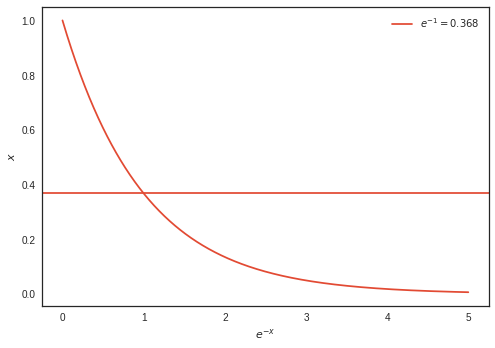# Plotting¶

## Unit 6, Lecture 1¶

Numerical Methods and Statistics

# Lecture Goals¶

1. Be able to add titles, axes labels, and legends to plots
2. Know about the different plotting types (points, lines)
3. Be able to choose which plot is most appropiate for the probability distributions
4. Be able to put formatted LaTeX into plot elements

# Plotting Probability Distributions¶

Let's plot the probability distributions we just learned. First we have to import our tools:

In :
%matplotlib inline
#the line above is for ipython notebooks only - makes plots appear in our notebook
import matplotlib.pyplot as plt #we import a sub-module called pyplot and call it plt for short
import numpy as np #import numpy


## Bernoulli Distribution¶

The Bernoulli has a state space of 2, so this would be best suited to a bargraph. Bargraphs are good to use when the state space is discrete and small.

In :
p = 0.8
bar_loc = [0,1]
height = [1 - p, p]
plt.bar(bar_loc, height)
plt.show()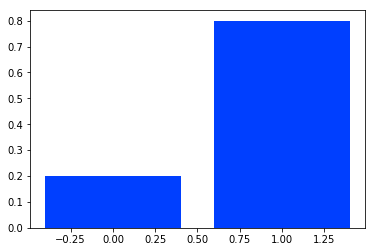The x-axis is a little strange though; the labels don't really make sense. You can set them via a list in fact:

In :
labels = ['failure', 'success']
plt.bar(bar_loc, height, tick_label=labels)
plt.show()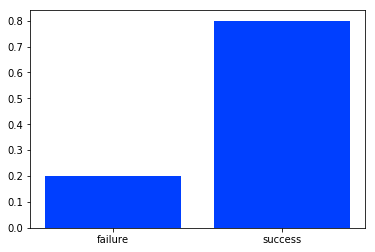Better!

## Geometric Distribution¶

The geometric distribution is discrete and can go from 1 to $\infty$

In :
plt.style.use('seaborn-bright')
n = np.arange(1, 25)
p = 0.2
pn = (1 - p)**(n - 1) * p

plt.plot(n, pn)
plt.show()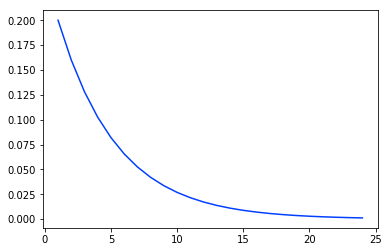There is a problem with this graph - it appears the the distribution is continuous and it starts at 0. We change this by using matplolib's line styling strings:

In :
plt.plot(n, pn, 'o')
plt.show()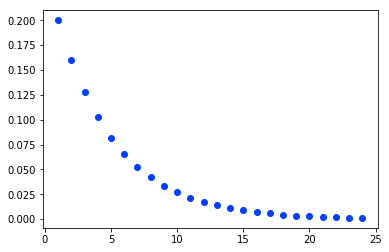The 'o' makes it so little os appear. There are other characters as well, like '+'. '-' makes a line. You can mix them too:

In :
plt.plot(n, pn, '-o')
plt.show()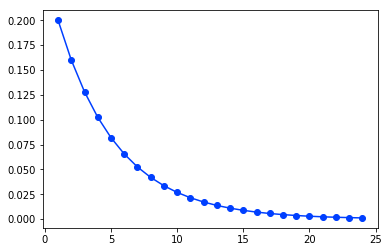There are also color codes, which are single letters that go before the marking specifier

In :
plt.plot(n, pn, 'bo')
plt.plot(n, pn, 'r-')
plt.show()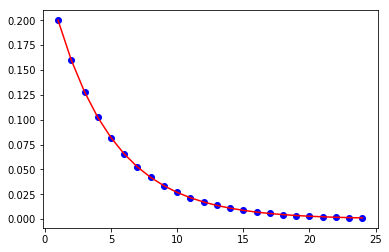To adjust the axis limits, use these functions:

In :
plt.plot(n, pn, 'bo-')
plt.xlim(1, 20)
plt.show()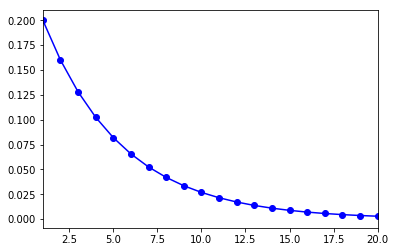Sometimes you don't want to squish all the color and marker codes together. You can specify separately like so:

In :
plt.plot(n, pn, marker='o', linestyle=':')
plt.xlim(1, 20)
plt.show()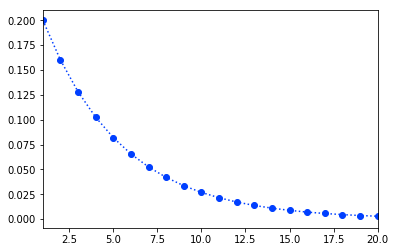## Binomial Distribution¶

The binomial distribution is discrete and goes from $0$ to $N$. It's the number of successes in $N$ trials.

In :
plt.style.use('ggplot')
from scipy.special import comb
N = 10
p = 0.3
n = np.arange(0, N+1)
pn = comb(N, n) * p**n * (1 - p)**(N - n)

plt.plot(n, pn, 'ro-')
plt.show()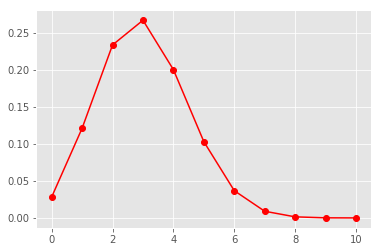Notice that comb is a scipy function, so it knows how to deal with arrays. To get the same thing without using arrays, we would have to use a for loop

## Poisson Distribution¶

The Poisson distribution is often an approximation to a binomial distribution. For example, let's say we're playing the lottery and 650 million people have a ticket. The odds of winning are 1 in 300 million.

$$\mu = Np = \frac{650}{300}$$

The sample space is $0$ to $\infty$ and it is discrete.

In :
from scipy.special import factorial
mu = 650 / 300.
n = np.arange(0, 10)
pn = np.exp(-mu) * mu**n / factorial(n)

plt.plot(n, pn, 'yo-')
plt.show()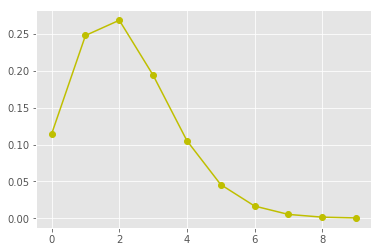# Further Plotting Details¶

## Adding LaTeX¶

As you saw in recitation you may add LaTeX

In :
plt.style.use('seaborn-notebook')
plt.plot(n, pn, marker='o', linestyle='-', color='orange')
plt.title('Poisson Distribution')
plt.xlabel('$x$')
plt.ylabel('$P(X = x)$')
plt.show()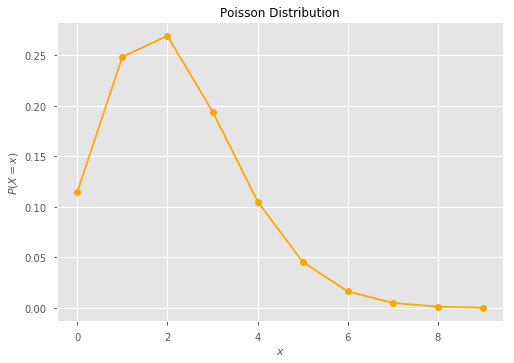In :
plt.plot(n, pn, marker='o', linestyle='-', color='orange')
plt.title('Poisson Distribution')
plt.xlabel('$x$')
plt.ylabel(r'$P(x) = \frac{e^{-\mu} x^\mu}{\mu!}$')
plt.show()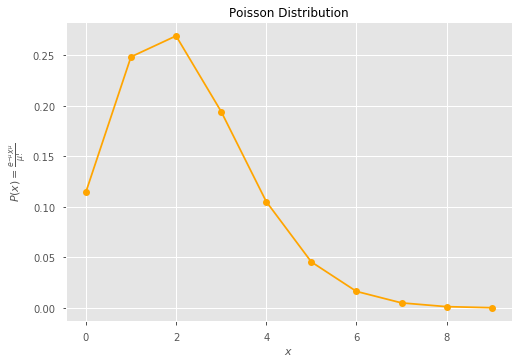## Adding Vertical/Horizontal Lines¶

In :
plt.axvline(x=2)
plt.axhline(y=0.15, color='blue')
plt.plot(n, pn, 'yo-')
plt.show()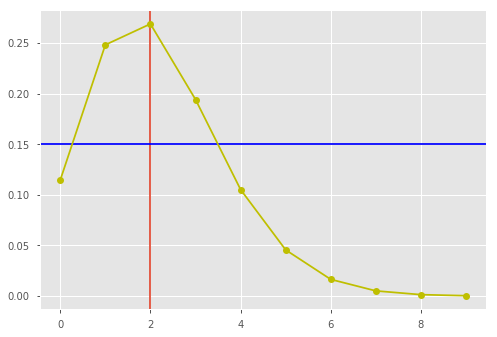# Creating Legends¶

Legends can be automatically placed

In :
plt.style.use('seaborn-white')
n = np.arange(1, 25)
p = 0.2
Pn = (1 - p)**(n - 1) * p

plt.plot(n, Pn, label='Geometric Distribution', marker='o', linestyle='--')
plt.axvline(x=2, label='$n=2$', color='blue', linestyle=':')
plt.xlim(1,24)

plt.legend()
plt.show()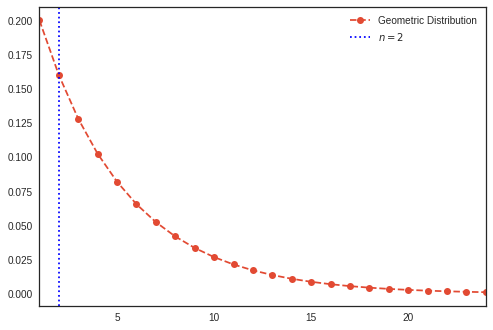In :
n = np.arange(1, 25)
p = 0.2
Pn = (1 - p)**(n - 1) * p

plt.plot(n, Pn, label='Geometric Distribution', marker='o', linestyle='--')
plt.axvline(x=2, label='$n=2$', color='blue', linestyle=':')
plt.xlim(1,24)

plt.legend(loc='center')
plt.show()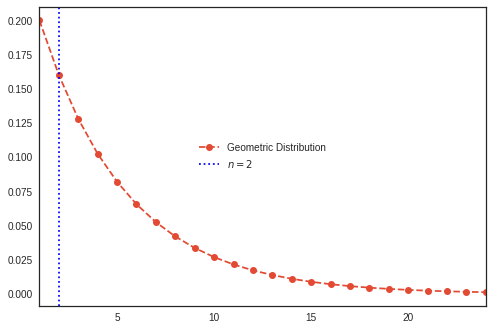One of the nice features of labels is that you can use the result of your calculation to be a label

In :
expected_value = 1 / p

plt.plot(n, Pn, label='Geometric Distribution', marker='o', linestyle='--')
plt.axvline(x=expected_value, label='$E[n] = {:.2f}$'.format(expected_value), color='blue', linestyle=':')
plt.xlim(1,24)

plt.legend(loc='center')
plt.show()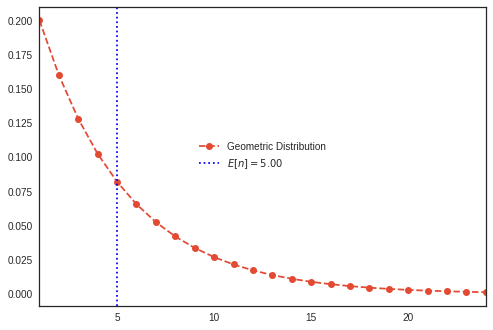You'll notice that the $E$ is italicized. That's because LaTeX treats it as a variable. We can get it to look normal by just taking it out of the enclosing $ In : plt.plot(n, Pn, label='Geometric Distribution', marker='o', linestyle='--') plt.axvline(x=expected_value, label='E$[n] = {:.2f}$'.format(expected_value), color='blue', linestyle=':') plt.xlim(1,24) plt.legend(loc='center') plt.show()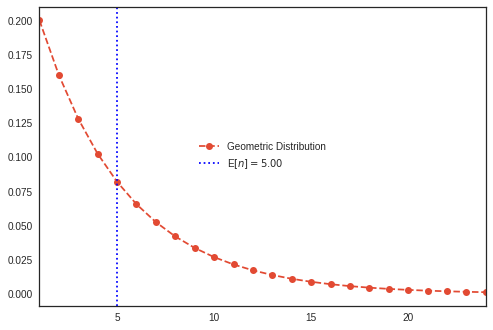# LaTeX String Mangling¶ You'll notice that the curly braces can be used for two things: Python string formatting OR LaTeX operators. Sometimes this can lead to conflicts: In : x = np.linspace(0,5, 1000) y = np.exp(-x) plt.plot(x, y) plt.ylabel('$x$') plt.xlabel('$e^{-x}$') plt.axhline(y=np.exp(-1), label='$e^{-1} = {:.3f}$'.format(np.exp(-1))) plt.legend() plt.show()  --------------------------------------------------------------------------- KeyError Traceback (most recent call last) <ipython-input-23-f75ceb63b756> in <module>() 4 plt.ylabel('$x$') 5 plt.xlabel('$e^{-x}$') ----> 6 plt.axhline(y=np.exp(-1), label='$e^{-1} = {:.3f}$'.format(np.exp(-1))) 7 plt.legend() 8 plt.show() KeyError: '-1'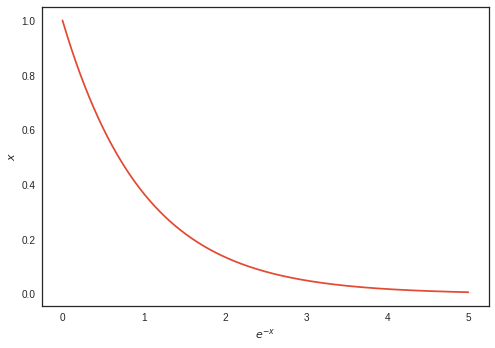To get a curly brace past Python, just double-up: In : x = np.linspace(0,5, 1000) y = np.exp(-x) plt.plot(x, y) plt.ylabel('$x$') plt.xlabel('$e^{-x}$') plt.axhline(y=np.exp(-1), label='$e^{{-1}} = {:.3f}\$'.format(np.exp(-1)))
plt.legend(loc='best')
plt.show()